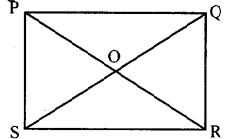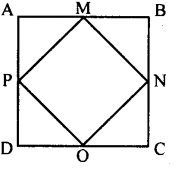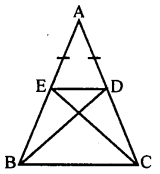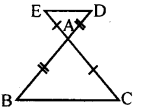# KSEEB Solutions for Class 8 Maths Chapter 11 Congruence of Triangles Ex 11.2

In this chapter, we provide KSEEB SSLC Class 8 Maths Chapter 11 Congruence of Triangles Ex 11.2 for English medium students, Which will very helpful for every student in their exams. Students can download the latest KSEEB SSLC Class 8 Maths Chapter 11 Congruence of Triangles Ex 11.2 pdf, free KSEEB SSLC Class 8 Maths Chapter 11 Congruence of Triangles Ex 11.2 pdf download. Now you will get step by step solution to each question.

### Karnataka State Syllabus Class 8 Maths Chapter 11 Congruence of Triangles Ex 11.2

Question 1.
In the adjoining figure PQRS is a rectangle. Identify the congruent triangles formed by the diagonals.Δ PSR ≅ Δ PQR, Δ POQ ≅ Δ SOR
Δ SPQ ≅ Δ SRQ, Δ POS ≅ Δ QOR

Question 2.
In the figure ABCD is a square. M, N, O & P are the midpoints of sides AB, BC, CD and DA respectively. Identify the congurent triangles.Δ APM ≅ Δ BMD ≅ Δ CNO ≅ Δ DOP

Question 3.
In a triangle ABC, AB = AC. Points G on AB and D on AC are such that AE = AD. Prove that triangles BCD and CBE are congruent.In the figure AB = AC (data)
∴∠ABC = ∠ACB
[Base angles of an isosceles triangle]
AB = AC(data)
AB – AE = AC – AD (Axiom 3)
BE = DC
In Δ BCD and Δ CBE
∠BCD = ∠EBC
BE = DC (Proved)
BC = BC (Common side)
∴ Δ BCD= Δ CBE [SAS postulate]

Question 4.
In the adjoining figure the sides BA and CA have been produced such that BA = AD and CA = AE prove that DE || BC (hint : use the concept of alternate angles.)Δ AED and Δ ABC
AE = AC (data)
[Vertically opposite angles]
∴ Δ AED = ABC
[SAS postulate]
∴ ∠AED = ∠ACB [Congruent triangle property]
But these are alternate angles.
∴ DE || BC
Consequences of SAS postulate.

All Chapter KSEEB Solutions For Class 8 maths

—————————————————————————–

All Subject KSEEB Solutions For Class 8

*************************************************

I think you got complete solutions for this chapter. If You have any queries regarding this chapter, please comment on the below section our subject teacher will answer you. We tried our best to give complete solutions so you got good marks in your exam.

If these solutions have helped you, you can also share kseebsolutionsfor.com to your friends.

Best of Luck!!